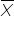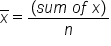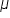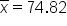+
Calculating the Center of Data

# Calculating the Center of Data

Author: Sophia Tutorial
##### Description:

Calculate mean, median, and mode from a given data set.

(more)

Sophia’s self-paced online courses are a great way to save time and money as you earn credits eligible for transfer to many different colleges and universities.*

No credit card required

37 Sophia partners guarantee credit transfer.

299 Institutions have accepted or given pre-approval for credit transfer.

* The American Council on Education's College Credit Recommendation Service (ACE Credit®) has evaluated and recommended college credit for 33 of Sophia’s online courses. Many different colleges and universities consider ACE CREDIT recommendations in determining the applicability to their course and degree programs.

Tutorial
what's covered
In this lesson, we’ll discuss determining the center of data. By the end of the lesson, you will understand how the mean, median, and mode provide useful information about data. You will also learn how to determine what the mean, median, and mode are for a variety of different data types. This lesson covers:
1. The Experimental Method
2. Measures of Center
3. Mean
4. Median
5. Mode
6. When to Use a Measure of Center

## 1. The Experimental Method

As a quick refresher, the fifth step in the experimental method is to analyze the results of a test. This is to determine what the results tell you about the cause-and-effect relationship between two variables.

Step 5: Analyze the results of the test to determine what the results tell you about the cause and effect relationship between the two variables.

IN CONTEXT

Imagine you hypothesize that watering a tree causes it to grow. To test this, you plant two trees. You water one of the trees regularly and the other less often to see if there’s a difference in growth between the two. After a while, you look at the results to determine what they tell you about the cause-and-effect relationship of watering trees.

## 2. Measures of Center

When analyzing data, it’s important to know what the center of a data set is. This helps you summarize and organize the data set so you can convey something meaningful with that data. There are three measures of center that you’ll look at: mean, median, and mode. The mean is the average value for a data set; the median is the middle value, where half of the values are larger and half are smaller; and the mode is the value that occurs most often.

terms to know
Mean (x-bar)
The average value for a data set
Median
The middle value of a data set
Mode
The most common value in a data set

## 3. Mean

Mean and average are the same thing. Given a list of numbers, find the mean by adding up all the numbers and then dividing them by how many numbers there actually are.

The mean could be determined using a formula, as shown below. If the mean comes from a sample, it’s denoted by a symbol called "x bar," which is nothing more than an x with a straight line right above it:formulaThis x represents the values for your observations, and n represents the number of observations. This is how mean is calculated.

If the mean comes from a population, however, we use a Greek letter, mu:In general, Greek letters are used as symbols for quantities that come from population whereas Latin letters are generally used for quantities that come from a sample.

IN CONTEXT

Say you have a football team, and you want to figure out the average, or mean, height of the players. To do this, you draft a list of all the football players by height in inches.
76, 77, 74, 72, 71, 75, 78, 76, 79, 73, 72
How would you figure out what that mean’s going to be? Add up all the numbers; this is the sum of x. For this scenario x = 823. Divide that by the 11 observations. The mean height is approximately 74.82 inches.

Had this been a sample of 11 players from a team of 45, the mean would have been denoted by x bar, or.

## 4. Median

To find the median in a list of numbers, first arrange those numbers from smallest to largest. The median is the middle number on your list. If you happen to have an even number of values, simply add the two middle numbers in the list and divide them by two to get the median.

Given a list of ten different numbers, you could simply cross off the first four and the last four:

color:red;> 2, 4, 8, 12, 16, 20, color:red>24, 26, 30, 36

16+20=36
36/2=18

The median is between 16 and 20, so you add them together to figure out their average. They equal 36, which, divided it by 2, makes the median 18.

If you have an odd number of observations, you can cross off the numbers to the left and the numbers to the right.

color:red;> 3, 5, 9, 11 color:red>19, 21, 25

Here the middle number is 11, and this is the median.

IN CONTEXT

A teacher with 15 students gave an exam, and the scores were:

84, 68, 47, 63, 90, 95, 97, 81, 86, 100, 72, 92, 77

Clearly the numbers are not in any particular order.

How do you determine the median?

You need to sort the numbers from smallest to largest. Then you can cross off the numbers to the left and the numbers to the right until you reach the middle.

color:red;> 47, 63, 68, 72, 75, 77, 78, 81 color:red>84, 86, 90, 92, 95, 97, 100</span> </span>

You wind up with your median, which is 81. This means that seven of the 15 students earned less than 81%, and seven earned better than 81% on the exam.

Suppose the teacher actually forgot that there was a 16th student, and that student happened to get an 85%. You now have an even number of students. How do you determine the median in this case?

Simply reorder the numbers and figure out the average between that eighth and ninth number.

84, 68, 47, 63, 90, 95, 97, 81, 86, 100, 72, 78, 75, 92, 77, 85

The eighth number is 81, and the ninth number is 84. To get the average, add them together and divide by 2. The median here is 82.5%. This means half the class did better than 82.5%, and half the class did worse.

## 5. Mode

If you have a list of values, the mode is the value that occurs most often on that list. To find the mode, simply count how often each value occurs. It’s possible to have more than one mode, if two or more different values appear equally most often, or no mode if none of the values repeat.

IN CONTEXT

Say you decide to survey 19 households in a neighborhood to see how many cars each household has. Nine of the households have two cars each, while two households don't have any. Four of them only have one car each. Three of them actually have three, and one household has four cars. The mode is the value that occurs most often, so you can see that the mode in this case is two cars.## 6. When to Use a Measure of Center

Measures of center provide you with important information about a data set. They tell you where the middle is located or what a typical value in a data set looks like. However, each measure of center is best for different types of data

With nominal data, such as gender, the mode is the correct measure of center to use, because the only information you can use is how often each value occurs.

For ordinal data, such as first, second, third, et cetera, the median is the correct measure of center. This is because ordinal data can be put in order, and you can find the middle value by arranging the data from smallest to largest.

hint

``` Make sure to arrange the numbers in order when working with ordinal data. Be careful not to simply select the middle value of the numbers before arranging, because this might not be the correct value.
```

For interval or ratio data either the median or the mean can be used as a measure of center. The mean could be used because the data consists of numbers, while the median could be used because we can arrange the numbers from smallest to largest.

big idea

<caption>Measures of Center</caption>
 Nominal Data Ordinal Data Gender Mode 1st, 2nd, 3rd,... Median 45 Degrees Median or Mean Twice as large Median or Mean

summary
This lesson reviewed the experimental method and how it relates to the measures of center. There are three measures of center. The mean is the average of a data set. The median is the middle value of a data set. Lastly, the mode is the value that occurs most often. You also looked at when to use a measure of center, because some situations are more appropriate for a certain type of measure.

Source: This work is adapted from Sophia author Dan Laub.

Terms to Know
Mean (x-bar)

The average value for a data set

Median

The middle value of a data set.

Mode

The most common value in a data set.

Formulas to Know
MeanRating